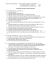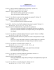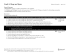C Geometry Name: 1.4 Worksheet – Constructing Parallel and

Transcription

C Geometry Name: 1.4 Worksheet – Constructing Parallel and
Geometry
Name:________________________
1.4 Worksheet – Constructing Parallel and Perpendicular Lines
1. Given AB , use the midpoint construction to construct a perpendicular bisector. Label the
intersection, label congruent parts on the diagram, and list one pair of congruent or equal pieces.
A
A
B
B
Segments: _______ ≅ __________
Angles: ___________ = ____________
2. Construct the perpendicular line THROUGH A POINT ON THE LINE. Work backwards from
the midpoint construction.
A
C
3. Construct the perpendicular line THROUGH A POINT not on THE LINE. Work backwards
through the midpoint construction.
B
A
4. Given ∠ABC, can you think of a way to create a line parallel to AB through point C?
A
B
C

5. Create a parallel line to DE through point F.
E
D
F
5. Construct a line parallel to the given line and through the given point.
6. Given sides of a rectangle. Construct the rectangle. (Hint: We need perpendicular lines
through A and through B).
A
B
7. If you are told that MN is the perpendicular bisector of BC where point M is on BC . Draw the
diagram and completely label it with all known relationships.
8. If you are constructing the perpendicular line through point A (A is on the line), determine the next step.
Step #1 – Place compass at point A, and create two intersections B & C on either side of point A.
Step #2 – Place compass pointer at point B and extend its measure beyond A and make an arc above
and below point A.
Step #3 -- __________________________________________________________________________
9.
COPYING A SEGMENT
Given AB, CD, & EF . Use the
copy segment construction to
create the new lengths listed
below.
A
C
E
B
D
F
2.5CD
CD + 1.25AB
10. Construct an angle bisector for the given angle. Label the diagram with the congruent parts as a result of
the construction.

Geometry Secondary Education MAFS.912.G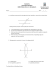7.3 Finding Volume Using Cross Sections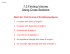Perpendicular bisectors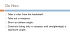1 In the diagram below of circle O, , and . Which statement must be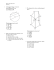STUDY GUIDE for Unit 1 Test Geometry 1. Z?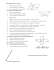GR-9T2-MA-CAPS-3-5-Cons-Geom-Fig-1-Revise - E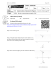Linear Equations negative one. Non Vertical Lines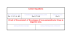Solve the problems below to answer the riddle: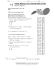1-2 Practice A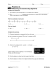Algebra with shapes How to . . .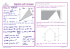G.SRT.4 Wkst 1B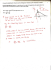The Midpoint Formula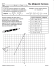Pre-AP Algebra 2 Summer Review Worksheet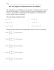G.C.2 Wkst 5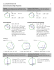4.5 Equations of Parallel and Perpendicular Lines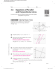The Sieve of Eratosthenes - E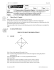Coordinate Geometry Unit Test Review